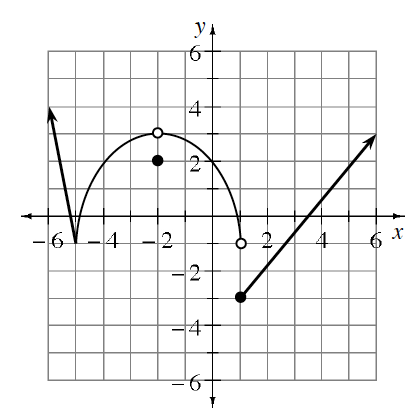### Home > PC3 > Chapter 9 > Lesson 9.1.4 > Problem9-85

9-85.This problem is a checkpoint for evaluating limits from graphs and tables.

1. Use the piecewise-defined function, $f$, graphed at right to evaluate each of the limits below.

1. $\lim\limits_{x \to -5}f(x)$

1. $\lim\limits_{x \to -2^-} f ( x )$

1. $\lim\limits_{x \to 1} f ( x )$

1. $\lim\limits_{x \to 1^+} f ( x )$1. Let $f(x)=\frac { 4 ^ { x } - 3 ^ { x } } { x }$. Copy and complete the table of values below to estimate the value of $\lim\limits_{x \to 0} \frac { 4 ^ { x } - 3 ^ { x } } { x }$.

 $x$ $–0.01$ $–0.001$ $–0.0001$ $0.0001$ $0.001$ $0.01$ $f(x)$